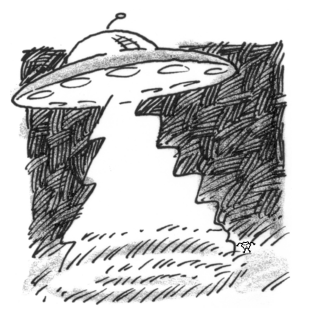### Home > APCALC > Chapter 7 > Lesson 7.1.5 > Problem7-48

7-48.

An alien uses mysterious powers to make crop circles in a Nebraska wheat field. If the radius of a crop circle increases at the rate of $6$ ft/min, calculate the rate at which the circle’s area is increasing when the radius is $150$ feet.

Start with the geometric equation of the area within a circle. There is no Calculus involved in this step.
$A = πr^²$
Do NOT substitute a value for $r$, because the $r$ changes. That is where Calculus comes in...

Implicitly differentiate with respect to time, $t$.

$\text{You will see both }\frac{dA}{dt}\text{ and }\frac{dr}{dt}\text{ in the differential equation.}$

$\frac{dA}{dt},\text{ of course, will give you the answer to this problem.}$

$\text{You can now solve for }\frac{dA}{dt},\text{ the rate the area was changing when the radius was 150 ft.}$

$\text{Evaluate the differential equation at }r=150\text{ and }\frac{dr}{dt}=150.$

$\frac{dA}{dt}=1800\pi \frac{\text{ft}^{2}}{\text{min}}$